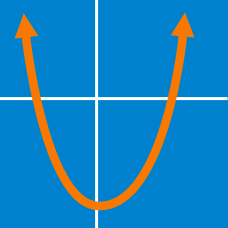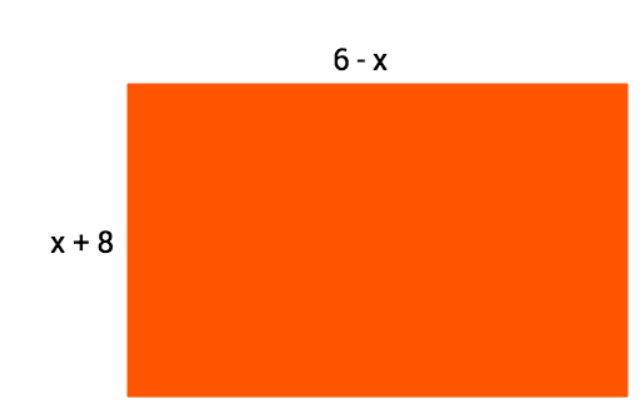Algebra

Challenge Quizzes

If $(x + 4)(3x - 1) = ax^2 + bx + c,$ what is the value of $a + b + c?$

The area of a rectangle can be expressed as $(2x^2 + 11x - 21).$ If the width of the rectangle is $(x + 7),$ which expression could represent the length?

If $bx^2 + 32x = 4x(x - a),$ what is the value of $ab\, ?$

The Smith family has $2,581 \text{ft}^2$ available in their rectangular backyard to put in a swimming pool. They plan to use the entire space. If the pool will have dimensions of $20 \text{ft}$ by $80 \text{ft}$, and they want a patio of equal width around the pool, what will be the width of the patio, in feet?What is the maximum possible area for the rectangle shown?

×